Student Resources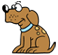Don't want

Big Ideas Math: Blue

Chapter 1: Solving Equations (pp. 1 - 45)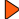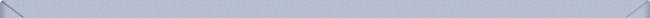Math Tool Paper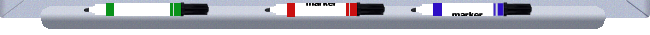Chapter 2: Graphing Linear Equations and Linear Systems (pp. 46 - 103)Math Tool PaperChapter 3: Writing Linear Equations and Linear Systems (pp. 104 - 145)Math Tool PaperChapter 4: Functions (pp. 146 - 181)Math Tool PaperChapter 5: Angles and Similarity (pp. 182 - 227)Math Tool PaperChapter 6: Square Roots and the Pythagorean Theorem (pp. 228 - 271)Math Tool PaperChapter 7: Data Analysis and Displays (pp. 272 - 309)Math Tool PaperChapter 8: Linear Inequalities (pp. 310 - 347)Math Tool PaperChapter 9: Exponents and Scientific Notation (pp. 348 - 395)Math Tool PaperAdditional Topics : (pp. 396 - 403)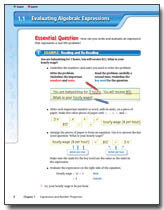Math Tool PaperAppendix A: My Big Ideas Projects (pp. A1 - A9)Support Links• Swiss Family Robinson: PDF   AudioAppendix B: Financial Literacy (Online Only)Math Tool Paper